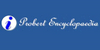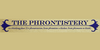Minuend• (n.) The number from which another number is to be subtracted.
Found on http://thinkexist.com/dictionary/meaning/minuend/

minuendnoun the number from which the subtrahend is subtracted
Found on https://www.encyclo.co.uk/local/20974

MinuendMin'u·end noun [ Latin minuendus to be diminished, from minuere to lessen, diminish. See Minish .] (Arith.) The number from which another number is to be subtracted.
Found on http://www.encyclo.co.uk/webster/M/71

MinuendA number from which another number is to be subtracted. See also: Subtraction, Subtrahend.
Found on http://www.diracdelta.co.uk/science/source/m/i/minuend/source.html

MinuendIn mathematics, a minuend is the number from which another number (known as the subtrahend) is to be subtracted.
Found on http://www.probertencyclopaedia.com/browse/GM.HTM

minuendnumber from which another is to be subtracted
Found on http://phrontistery.info/m.html

minuend[n] - the number from which the subtrahend is subtracted
Found on http://www.webdictionary.co.uk/definition.php?query=minuend
No exact match found